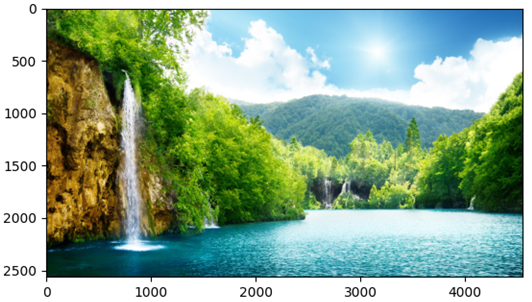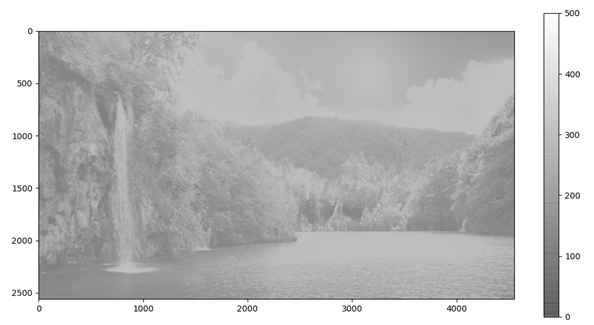# How to Display, Modify and Save Images in Matplotlib

4/5 - (1 vote)

This article will show you how to visualize, modify and save images in Python, more specifically in Matplotlib. In the following sections, we will see how to load images, how to modify some of their properties and finally how to save them.

## Long story short

If you want to import an image and to display it in a Matplotlib window, the Matplotlib function imread() works perfectly. After importing the image file as an array, it is possible to create a Matplotlib window and the axes in which we can then display the image by using imshow(). By changing some of the properties available within imshow() we can  vary the color, the size and even decide to crop the displayed image. Once we are satisfied with the result from our modifications, the function savefig() allows to save the figure by specifying as input parameters the path in which we want the image to be stored. Exploiting some additional parameters of this latter function it is possible to decide whether to make the borders of the image transparent or even to delete them.

## Importing an Image into Your Script

We start the tutorial by importing the image in our script, in this way we will be able to take further actions and hence modify its appearance. To do this, we exploit the Matplotlib function imread(), which reads an image from a file into an array (official documentation: https://matplotlib.org/stable/api/_as_gen/matplotlib.pyplot.imread.html).

The function takes as the only input parameter the path of the image file that we want to import.

The image file is converted into a M x N x 3 (in the case of a RGB image) array with M and N standing for the number of pixels for the width and height, respectively. In the case of a RGB image, each MiNj subarray will contain 3 numbers corresponding to the combination of Red Green Blue values that identify that specific color; in the case of RGBA images, we would have a M x N x 4 array.

In the following code lines, we import the Matplotlib library and we assig to the variable “pic” the array describing the image file that we wanted to import.

```import matplotlib.pyplot as plt

url = r"file_path"
print(pic)
```

The result of the printing command will display in your terminal a 3D array describing the image file that you uploaded.

## Obtaining the Image Size

It is possible to know how many pixels compose our image and what type of color code it uses, by just applying the method .shape() to the variable in which we stored the array describing the image file. Indeed, by getting the array shape, we will obtain the different dimensions of the array, since each subarray will represent a pixel with its color values, we will get an indication of the exact number of pixel that make up our image (i.e. each element corresponds to a pixel).

```# get image pixels
print(pic.shape)
>> (2559, 4550, 3)
```

In our case, we have an image that is 2559 pixels high, 4550 pixels wide and is defined according to a RGB color scheme (from the 3 values in the last array dimension).

## Display Images in Matplotlib

In this section we will see how to display an image file in a matplotlib window; the procedure is extremely easy and really similar to the one that is used for plotting a normal graph. We start by creating the matplotlib figure and the axes.

```# display the image in a mpl figure
fig = plt.figure()
ax = fig.subplots()
```

At this point, we use the Matplotlib function imshow() to display the image within the just created axes. The imshow() function accepts as its main input parameter the variable referring to the image file, which in our case, is “pic”. The result from the following code lines is then displayed in Figure 1.

```ax.imshow(pic)
plt.show()
```Figure 1: After creating a matplotlib figure and the axes, imshow() allows displaying the image file within the axes of the figure.

## Changing the Properties of the Displayed Image

By specifying other input parameters within the imshow() function it is possible to modify some of the properties of the displayed image, like the size, the color and its transparency.

To be able to modify the just mentioned properties, we have to index the pixels on which we want to apply any changes, if we want to modify the entire image, we just have to index all the pixels (in this case, this means writing pic[:, :, 1]). The first property that we will change is the transparency of the image; we can do this by changing the value of the alpha parameter (from to 0 to 1).

We can also change the color map that is used in the image, for example using a “gray” color map will turn our color image into a black and white picture. This thing can be done by modifying the parameter cmap (here you can find all the possible color maps available for Matplotlib: https://matplotlib.org/stable/tutorials/colors/colormaps.html).

If we want to change the color range used within the selected color map, we could modify the values of the parameters vmin and vmax for tuning the highest and lowest values of the color range. If we don’t specify these two parameters, the whole color range will be used for displaying the image.

In addition to changing the color properties, we can also modify the aspect ratio of the image pixels; this is an important topic to keep in mind, especially if we want to change the size of the axes of the figure. This can be done by exploiting the parameter aspect; we can choose between two different options auto or equal. Using auto will keep the axes fixed and the aspect ratio of the pixels is adjusted accordingly, generally resulting in non-square pixels. On the other hand, equal ensures an aspect ratio of 1 for the pixels in the image, meaning square pixels.

You can find additional information about the imshow() function and all its properties in the official documentation page: https://matplotlib.org/stable/api/_as_gen/matplotlib.pyplot.imshow.html. In the following code line, we assign to the variable f the image file and we change all the above-mentioned properties.

`f = ax.imshow(pic[:, :, 1], alpha=0.5, cmap='gray',  vmin=0, vmax=500, aspect='auto')`

It is also possible to add a color bar next to the image by applying the method colorbar() to the figure.

`f = ax.imshow(pic[:, :, 1], alpha=0.5, cmap='gray',  vmin=0, vmax=500, aspect='auto')`

The final result is displayed in Figure 2; notice that since we set aspect = ‘auto’, the aspect ratio of the pixels has changed hence producing an image whose shape is slightly different (see the video for a better example) from the one in Figure 1.Figure 2: Changing the properties within the imshow() function allowed for changing the image color scale and pixel aspect ratio.

## Changing the Axes Range and Visibility

As you can see from the previous figures, all the axes and the tick labels are still present and visible in the plotting window. In the case we would like to remove them and hence have just the image plotted within the Matplotlib window, we can disable the axes by using .axis(‘off’). If we are also interested in plotting just a portion of the image, we can do it by adjusting the value of the maximum x and y values to be displayed on each of the axes. All these commands are displayed in the following code lines:

```# changing the axes properties
ax.set_xlim(0, 2500)
ax.set_ylim(2500, 0)
ax.axis('off')
```

You can notice that we chose to display the image for its entire height but with a width going from 0 to the value 2500. Also notice that the coordinates of the y-axis are given in the inverted order; this is because the y-axis of the image goes from the top to the bottom of the figure. If you gave the limits of the y-axis in the opposite order, the imaged will be flipped. The final result is displayed in Figure 3.Figure 3: It is possible to crop the image by just changing the values of the maximum x and y coordinates along the axes. In order to display only the image, it is then possible to set to “off” the axes visibility.

## Saving the Image File

Once we finished to edit our image, we can save it by using the Matplotlib function savefig(). The only mandatory input parameter is the path to which we want to save the image. In the case we want to save the image without any white border, we can specify the two optional parameters bbox_inches (setting it to “tight”) and pad_inches (setting it equal to zero). With the first option we make the border of the figure to adapt to the size of the image, with the second one we set to zero the amount of border around the figure (we first have to set to “tight” the property bbox_inches).

`plt.savefig(r"C:\Users\Andrea\Desktop\newimage.jpg", bbox_inches='tight', pad_inches=0)`

## Conclusions

In this article, we just learned how to import and modify an image file by using Matplotlib. First of all, we imported the image file within a Matplotlib window; then by using the appropriate functions, it was possible to display the image, change its color, its size and aspect ratio. In the end we also saw how to save the modified image into a new image file. All these procedures represent a really easy and fast solution for a quick adjustment of images within a Python session.

## Programmer Humor – Blockchain“Blockchains are like grappling hooks, in that it’s extremely cool when you encounter a problem for which they’re the right solution, but it happens way too rarely in real life.” source – xkcd